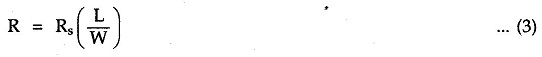# Integrated Resistors

## Integrated Resistors:

In Integrated Resistors circuit design, the importance is given to the maximum usage of transistors. For example digital CMOS, nMOS and GaAs circuits are fabricated entirely with transistors and diodes. The resistors are grouped into two groups ; one formed within monolithic IC and other composed of film resistors.

The monolithic IC resistors consist suitably dimensioned layers which would form part of the transistor normally. Obviously the resistivity of such layers is determined from transistor characteristics.

If the resistor is formed in one of the isolated regions of epitaxial layer during base or emitter diffusion, then it is called diffused resistor. It is very economical process as no additional steps in fabrication are needed. But the limitation of the diffused resistor is that the range of the value of resistance is very small.

For larger value of resistor, the larger area of silicon is required. Hence the high value resistances are realized by using pinch resistor as shown in the Fig. 1.21. The amount of silicon required for the value of resistor beyond 100 k K2 is relatively low: The accuracy of such resistors is poor. For high value resistors accuracy is not important point to take care. It consists of p base layer constricted by an n+ emitter layer, leading to an effective thickness equal to base thickness of a npn transistor.As we have already studied that the resistance can be realized by using a defined volume of semiconductor region. Consider a sheet of material with length L and width W as shown in the Fig. 1.22. Let t be the thickness and p be the uniform resistivity.

The resistance R between layers A and B is given byFor square surface area, W = L, then the resistance of the material is given byThus rearranging equation (1) using (2), we can writeHere ratio L / W  is called aspect ratio. Thus using this technique base resistor in

the range 20 Ω to 300  k Ω can be fabricated. Similarly the resistance of the emitter diffusion can be fabricated as sheet resistor but the range of resistance is only 10 to 1 k Ω.By using n-epitaxial collector layer, the large value resistances than base and emitter diffusion can be achieved. Such resistors are called epitaxial resistors as shown in the Fig. 1.23.